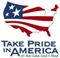Scientific Investigations Report 2007–5173

U.S. GEOLOGICAL SURVEY
Scientific Investigations Report 2007–5173

## Conversion Factors

### Inch/Pound to SI

Multiply By To obtain
Length
foot (ft) 0.3048 meter (m)
mile (mi) 1.609 kilometer (km)
Area
acre 0.4047 hectare (ha)
Volume
cubic foot (ft3) 0.02832 cubic meter (m3)
Flow rate
cubic foot per second (ft3) 0.02832 cubic meter per second (m3/s)
gallon per minute (gal/min) 0.06309 liter per second (L/s)
gallon per day (gal/d) 0.003785 cubic meter per day (m3/d)
Transmissivity
foot squared per second (ft2/s) 0.09290 meter squared per second (m2/s)

### SI to Inch/Pound

Multiply By To obtain
Length
millimeter (mm) 0.03937 inch (in.)
meter (m) 1.094 yard (yd)
Area
square meter (m2) 6.290 square foot (ft2)
Volume
cubic meter (m3) 6.290 barrel (petroleum, 1 barrel = 42 gal)
Flow rate
meter per second (m/s) 3.281 foot per second (ft/s)
millimeter per day (mm/d) 0.03937 inch per day (in/d)
Mass
kilogram (kg) 2.205 pound avoirdupois (lb)

Temperature in degrees Celsius (°C) may be converted to degrees Fahrenheit (°F) as follows:

°F=(1.8×°C)+32

Vertical coordinate information is referenced to the North American Vertical Datum of 1988 (NAVD 88).

Some vertical coordinate information is referenced to the now superseded National standard geodetic reference for elevation, the National Geodetic Vertical Datum of 1929 (NGVD 29).

Horizontal coordinate information is referenced to the North American Datum of 1983 (NAD 83).U.S. Department of the Interior | U.S. Geological Survey